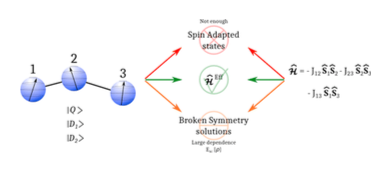Home | Highlights 2016

# Magnetic coupling constants in three electrons three centers problems from effective hamiltonian theory and validation of broken symmentry-based approaches

Magnetic coupling constants in three electrons three centers problems from effective hamiltonian theory and validation of broken symmentry-based approaches

D. Reta, I. de P.R. Moreira, F. Illas.
J. Chem. Theory Comput., 12 (2016) 3228.In the most general case of three electrons in three symmetry unrelated centres with S1 = S2 = S3 = 12 localized magnetic moments; the low energy spectrum consists of one quartet (Q) and two doublet (D1, D2) pure spin states. The energy splitting between these spin states can be described with the well-known Heisenberg-Dirac-Van Vleck (HDVV) model spin Hamiltonian, and their corresponding energy expressions are expressed in terms of the three different two-body magnetic coupling constants J12, J23, and J13. However, the values of all three magnetic coupling constants cannot be extracted using the calculated energy of the three spin-adapted states since only two linearly independent energy differences between pure spin states exist. In the present work we investigate the 3 electrons in 3 centres problem by means of ab initio effective Hamiltonian theory using MRCI wave functions and validate the broken symmetry approach to extract the J12, J23, and J13 in the general non-symmetric case.